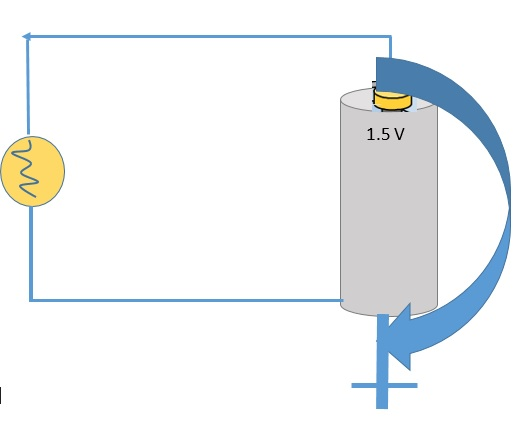# Introduction to Electric Energy and Power

Within an electric circuit as illustrated in the figure below, energy is supplied by a battery,
which uses energy from the potential energy (PE) stored in its chemical reactants.Whenever PE is lost, kinetic energy (KE) is gained by the electrons.
Subsequently, KE is converted to heat due to collisions with other electrons and atoms.
So, energy source ⇒ PE ⇒ KE ⇒ heat.
• P = εI = ΔεI
• ΔVI = IR
• P = (IR)R = I2R
• εI = I2R

### Exercises

Exercise-5 on Electric Energy and Power

Check your answers here: Solution to Exercise-5 on Electric Energy and Power

Exercise-6 on Electric Energy and Power

Check your answers here: Solution to Exercise-6 on Electric Energy and Power

Exercise-7 on Electric Energy and Power

Check your answers here: Solution to Exercise-7 on Electric Energy and Power